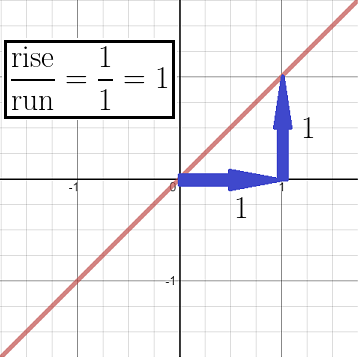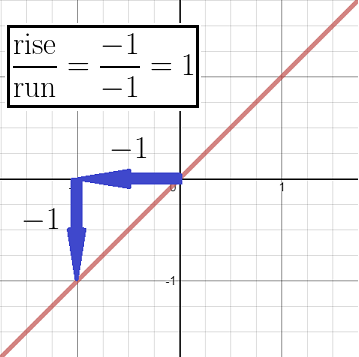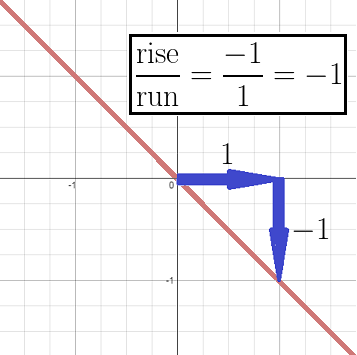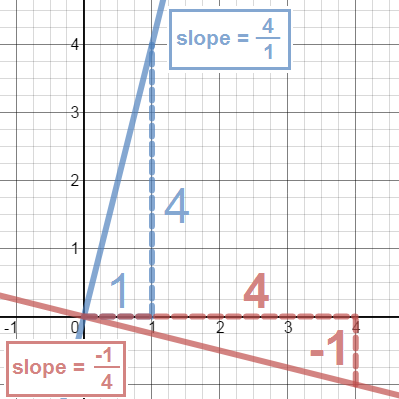# How to Find the Slope of a Perpendicular Line

Instructor: Flint Johnson

Flint has tutored mathematics through precalculus, science, and English and has taught college history. He has a Ph.D. from the University of Glasgow

In this lesson, we'll introduce the concept of slope and then discuss the relationship between the slopes of two perpendicular lines. Knowing this simple relationship will save you time on many math problems!

## What is Slope?

The short answer to this question is rise over run, but there is more to it than that. First of all, slope is a term you will see used in algebra because it tells us the angle of the line being graphed. A slope works like a fraction, with the rise, or up/down movement, over the run, or movement from side to side. If a line moved up one unit for every unit it moved to the right, it would have a slope of positive one.For that same line, you could think of it as going down one unit for every unit it moved to the left, and still get the same slope!If the sign of the rise and the run are the same, the line will have a positive slope. However, if the rise and run have opposite signs, then the slope will be negative.You could say that this line moves to the left while going up, or you could say that it moves to the right while going down. Either way, the signs of the rise and the run are always opposite, so the slope is always negative.

## Finding the Slope of a Perpendicular Line

Two lines are perpendicular if they intersect at an angle of exactly 90 degrees. If you know the slope of one of the lines, it's actually quite easy to find the slope of the other. This is because the slopes of any two perpendicular lines are negative reciprocals of one another. That may sound a bit confusing, so let's take a look at an example.Here, we have two lines that are perpendicular to each other. Using the graph, we can see that the blue lines goes up 4 units for every 1 unit it moves to the right. This means it has a slope of 4 over 1. The red line moves down one unit for every 4 units it moves to the right. This means it has a slope of -1 over 4. The fractions 4/1 and -1/4 are negative reciprocals of each other, meaning the numerators and denominators are swapped and the signs are opposite.

This rule holds true for all perpendicular lines and it can save you time on your math homework. For example, if you were asked to find the slope of a line perpendicular to another line that had a slope of -3/4, you wouldn't even need to graph it to find the answer. Using your knowledge of perpendicular lines, you could swap the numerator and denominator of the fraction and change the sign to find the slope of 4/3.

To unlock this lesson you must be a Study.com Member.

### Register to view this lesson

Are you a student or a teacher?